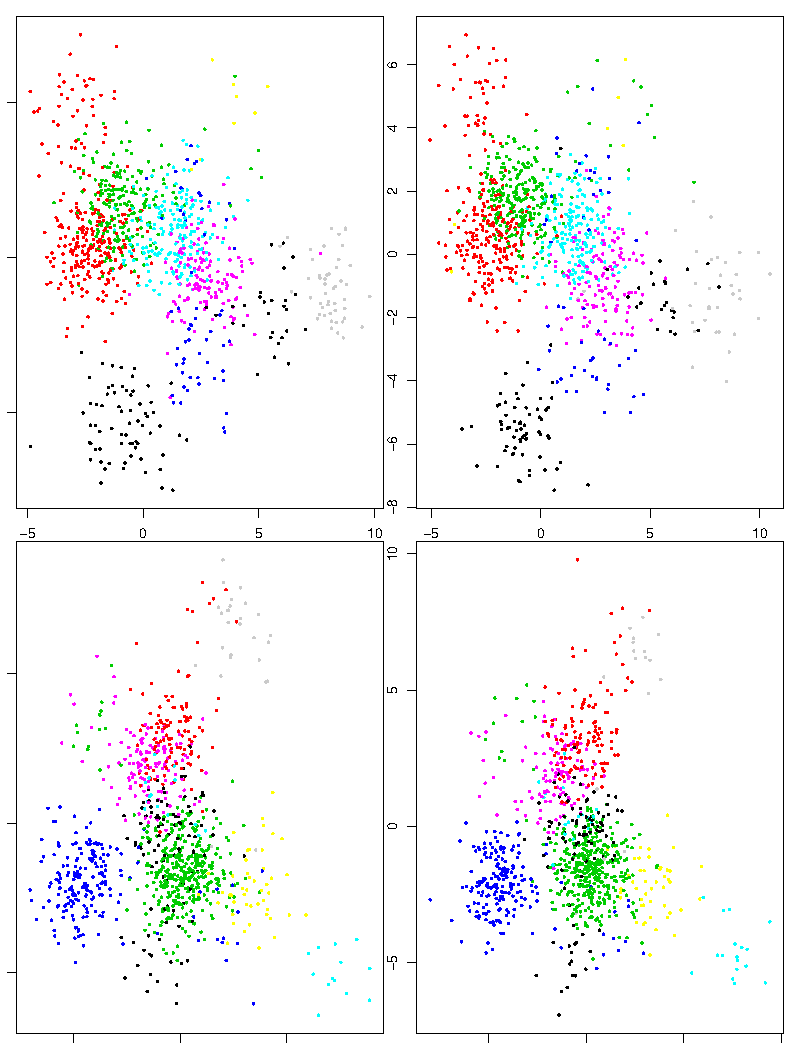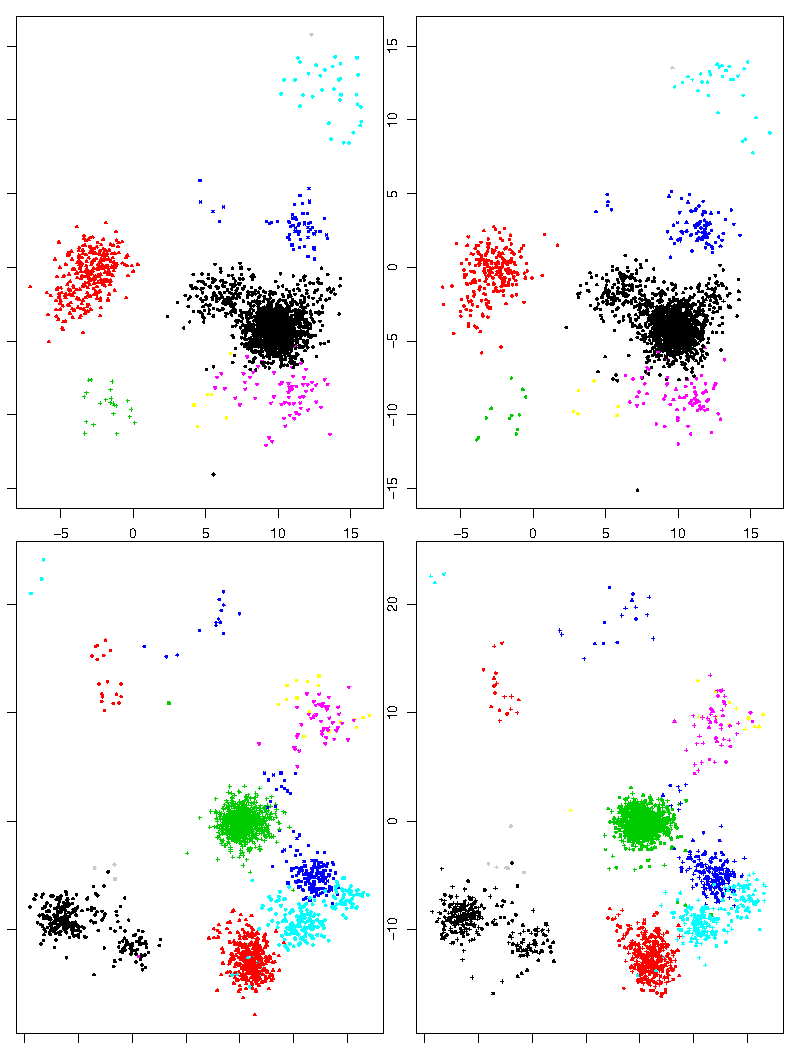# Cluster processes

This article Cluster processes was adapted from an original article by Peter McCullagh, which appeared in StatProb: The Encyclopedia Sponsored by Statistics and Probability Societies. The original article ([http://statprob.com/encyclopedia/ClusterProcesses.html StatProb Source], Local Files: pdf | tex) is copyrighted by the author(s), the article has been donated to Encyclopedia of Mathematics, and its further issues are under Creative Commons Attribution Share-Alike License.

2010 Mathematics Subject Classification: Primary: 62M20 Secondary: 62E99 [MSN][ZBL]

$\def\given{ {\,|\,}}$ $\def\E{ {\cal E}}$ $\def\K{ {\cal K}}$ $\def\N{ {\cal N}}$ $\def\R{ {\cal R}}$ $\def\S{ {\cal S}}$ $\def\T{ {\cal T}}$ $\def\U{ {\cal U}}$ $\def\Nat{ {\mathbb N}}$ $\def\upto{ {,\ldots,}}$ $\def\subdot{ {\hbox{\bf.}}}$

$\def\bfk{\vecf{k}}$ $\def\bfm{\vecf{m}}$ $\def\bfn{\vecf{n}}$ $\def\per{\mathop{\rm per}\nolimits}$ $\def\cyp{\mathop{\rm cyp}\nolimits}$ $\def\cof{\mathop{\rm cof}\nolimits}$ $\def\cov{\mathop{\rm cov}\nolimits}$ $\def\pr{\mathop{\rm pr}\nolimits}$ $\def\tr{\mathop{\rm tr}\nolimits}$ $\def\NJ{\mathop{\rm NJ}\nolimits}$ $\def\up{\uparrow}$ $\def\down{\downarrow}$ $\def\zero{ '''0'''}$ $\def\one{ '''1'''}$ $\def\booktitle#1{ ''#1''}$ $\def\vol#1{ '''#1'''}$ $\def\journal#1{ ''#1''}$

Cluster processes
Peter McCullagh University of Chicago

## Cluster processes

A $\R^d$-valued cluster process is a pair $(Y, B)$ in which $Y=(Y_1,\ldots)$ is an $\R^d$-valued random sequence and $B$~is a random partition of the index set~$\Nat$. The process is said to be exchangeable if, for each finite sample $[n]\subset\Nat$, the restricted process $(Y[n], B[n])$ is invariant under permutation $\sigma\colon[n]\to[n]$ of sample elements.

The Gauss-Ewens process is the simplest non-trivial example for which the distribution is as follows. First fix the parameter values $\lambda>0$, and the matrices $\Sigma^0, \Sigma^1$, both symmetric positive definite of order~$d$. In the first step, $B$~has the Ewens distribution with parameter~$\lambda$. Given~$B$, $Y$~is a zero-mean $\R^d$-valued Gaussian sequence with conditional covariances $\cov(Y_{ir}, Y_{js}\given B) = \delta_{ij} \Sigma^0_{rs} + B_{ij} \Sigma^1_{rs},$ where $\delta_{ij}$ is the Kronecker symbol. For $d=2$, a scatterplot colour-coded by blocks of the $Y$~values in $\R^2$ shows that the points tend to be clustered, the degree of clustering being governed by the ratio of between to within-cluster variances.Figure 1. The zero-mean Gauss-Ewens process in $\R^2$. The left box shows the first 1000 values colour-coded by block; the right box shows the next 1000 values using the same colour code. Each row is an independent realization with same parameter, so the blocks in row~1 are unrelated those in row~2. (eps)

For an equivalent construction we may proceed using a version of the Chinese restaurant process in which tables are numbered in order of occupancy, and $t(i)$~is the number of the table at which customer~$i$ is seated. In addition, $\epsilon_1,\ldots$ and $\eta_1,\ldots$ are independent Gaussian sequences with independent components $\epsilon_i\sim N_d(0, \Sigma^0)$, and $\eta_i \sim N_d(0, \Sigma^1)$. The sequence $t$ determines $B$, and the value for individual~$i$ is a vector $Y_i = \eta_{t(i)} + \epsilon_i$ in $\R^d$, or $Y_i = \mu + \eta_{t(i)} + \epsilon_i$ if a constant non-zero mean vector is included.

Each row of Fig. 1 is an independent realization of the Gauss-Ewens process with $\lambda=2$, $\Sigma^0=I_2$ and $\Sigma^1 = 9I_2$, with values colour-coded by block. In each row, the pattern of colours in the right panel matches closely that on the left, but the two rows are independent runs, so there is no relation between the blocks in the first row and those in the second.

Figure~2 is a similar illustration of the two-level tree-structured Gauss-Ewens process with two partitions $B'\le B$ representing classes and sub-classes. The parameters in this case are $\lambda=1$, $\Sigma^0=I_2$, $\Sigma^1 = 100 I_2$ and $\Sigma^2 = 5I_2$. The conditional distribution given $B, B'$ is zero-mean Gaussian with covariance matrix $\cov(Y_{ir}, Y_{js}\given B, B') = \delta_{ij} \Sigma^0_{rs} + B_{ij} \Sigma^1_{rs} + B'_{ij}\Sigma^2_{rs}.$ The sub-partition has the effect of splitting each main cluster randomly into sub-clusters.Figure 2. The two-level zero-mean Gauss-Ewens process in $\R^2$ colour-coded by major class. Each of the main blocks is partitioned at random into sub-blocks. The sub-partition is not illustrated, but can be inferred to some extent from the configuration. (eps)

In effect, each major cluster is an independent copy of the ordinary Gauss-Ewens process, so the configurations in each major cluster are random and independent with the same distribution.

## Classification using cluster processes

Despite the absence of class labels, cluster processes lend themselves naturally to prediction and classification, also called supervised learning. The description that follows is taken from McCullagh and Yang (2006) but, with minor modifications, the same description applies equally to more complicated non-linear versions associated using generalized linear mixed models (Blei, Ng and Jordan 2003). Given the observation $(Y[n], B[n])$ for the `training sample' $[n]$, together with the feature vector $Y_{n+1}$ for specimen $u_{n+1}$, the conditional distribution of $B[n+1]$ is determined by those events $u_{n+1}\mapsto b$ for $b\in B[n]$ and $b=\emptyset$ that are compatible with the observed partition. The assignment of a positive probability to the event that the new specimen belongs to a previously unobserved class seems highly desirable, even logically necessary, in many applications.

In the simplest version of the Gauss-Ewens model the between-blocks covariance matrix is proportional to the within-blocks matrix, i.e. $\Sigma^1 = \theta\Sigma^0$ for some scalar $\theta \ge 0$. The parameters in this reduced model are $\mu, \theta, \Sigma_0$, and an explicit analytic expression is available for the classification probability of a new unit such that $Y_{n+1} = y'$ as follows:

$$\pr(u_{n+1} \mapsto b \given Y[n+1], B[n]) \propto \begin{cases}\#b\; q_b(y' -\mu - w_b(\bar y_b - \mu)) &\quad b\in B[n]; \lambda \; q_\emptyset(y'-\mu) &\quad b=\emptyset. \end{cases}$$

Here, $\bar y_b$ is the mean for block~$b$, the weight $w_b = \theta\,\#b/(1+\theta\,\#b)$ is monotone increasing in block size, and $q_b(\cdot)$ is the density of the $d$-dimensional normal distribution with zero mean and covariance matrix $(1+w_b) \Sigma_0$. In practice, the parameters must first be estimated from the block means and within-blocks sample covariance matrix. If $\theta=0$, the $y$-values are irrelevant and the classification probabilities reduce to the Chinese restaurant process; otherwise, if $\theta > 0$, the conditional log odds $\log\left( \frac{\pr(u_{n+1} \mapsto b \given \cdots)} {\pr(u_{n+1} \mapsto b' \given\cdots)} \right)$ is the difference of two quadratic forms, which is linear in $y'$ if $\#b=\#b'$, and approximately linear otherwise. For classification purposes, this version of the Gauss-Ewens process may be viewed as a refinement of Fisher's linear discriminant model; it is more flexible in that the set of classes is not pre-specified, so that a new unit may be assigned with high probability to a previously unobserved class.

If the classes are tree-structured with two levels, we may generate a sub-partition $B'\le B$ whose conditional distribution given $B$ is Ewens restricted to the interval $[\zero_n, B]$, with parameter $\lambda'$. This sub-partition has the effect of splitting each main cluster or genus, randomly into sub-clusters representing species. For the sample $[n]$, let $t'(i)$ be the number of the sub-cluster or the name of the species to which individual~$i$ belongs. Given $B, B'$, the Gauss-Ewens two-level tree process illustrated in Fig. 2 is a sum of three independent Gaussian processes $Y_i = \eta_{t(i)} + \eta'_{t'(i)} + \epsilon_i$ for which the conditional distributions may be computed as before. In this situation, however, events that are compatible with the observation $B[n], B'[n]$ are of three types as follows: $u_{n+1}\mapsto b'\in B'[n],\quad u_{n+1}\mapsto \emptyset\subset b\in B[n],\quad u_{n+1}\mapsto \emptyset.$ In all, there are $\#B' + \#B + 1$ disjoint events for which the conditional distribution given the observation $B[n], B'[n], Y[n+1]$ must be computed. An event of the second type is one in which the new specimen belongs to the major class or genus~$b\in B$, but not to any of the species previously observed for this class. An event of the third type is a new genus.

## Acknowledgements

This is a revised version of parts of the article Random permutations and partition models from Lovric, Miodrag (2011), International Encyclopedia of Statistical Science, Heidelberg: Springer Science +Business Media, LLC. Support for this research was provided in part by NSF Grant DMS-0906592.

How to Cite This Entry:
Cluster processes. Encyclopedia of Mathematics. URL: http://encyclopediaofmath.org/index.php?title=Cluster_processes&oldid=37826